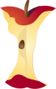Home > Grade Levels > Grade 5 >

## Generating Math Patterns From Rules#### Aligned To Common Core Standard:

Grade 5 Operations- 5.OA.3

### Printable Worksheets And Lessons

• Math Rule Tables Step-by-step Lesson- This is the real start of algebra. Replace the variables with the numbers.

• Guided Lesson - Read a graph and see how it relates to math rules. Find coordinates and see which rule matches up with the graph.

• Guided Lesson Explanation - I use visuals (a graph) to explain the first one, I hope you're a quick learner to grab and understand the two problems.

• Practice Worksheet - Five rule tables to complete and name the shape at the coordinates that are given.

• Matching Worksheet - Replace a variable and find the one that has the matching answer.

###Answer Keys

View Answer Keys- All the answer keys in one file.

###(Click Here to Upgrade)

#### Homework Sheets

I made sure that a nice solid example is provided for you. They are 2 operations mostly.

#### Practice Worksheets

These are two-sided problems. See if you can pass through them with ease.

#### Math Skill Quizzes

You are given a fixed variable. We would refer to these as plug and chug problems. Not sure if that is politically correct nowadays though.

 Quiz 1 Quiz 2 Quiz 3